• OFDM调制matlab仿真详细代码

万次阅读 多人点赞 2019-12-04 11:31:09
MATLAB仿真代码 % ================================================== ...% =================OFDM仿真参数说明：================ % % 子载波数 carrier_count ---200 % 总符号数 symbol_count ...

MATLAB仿真代码

% ==================================================
% 作者： 肆拾伍
% 时间：11/30 2019
% 版本：V3
% =================OFDM仿真参数说明：================
%
% 子载波数 carrier_count     ---200
% 总符号数 symbol_count      ---100
% IFFT长度 ifft_length       ---512
% 循环前缀 CP_length         ---512/4=128
% 循环后缀 CS_length         ---20
% 升余弦窗系数 alpha         ---7/32
% 调制方式                   QAM16、QPSK 可选
% 多径幅度 mult_path_am      ---[1 0.2 0.1]
% 多径时延 mutt_path_time    ---[0 20 50]
% ====================仿真过程=======================
% 产生0-1随机序列 => 串并转换 => 映射 => 取共轭、过采样
% => IFFT => 加循环前缀和后缀 => 加窗 => 并串转换 =>
% 多径信道 =>  加AWGN => 串并转换 => 去前缀 => FFT =>
% 下采样 =>  解映射 => 求误码率
% ==================================================
clear all;
close all;
carrier_count = 200; % 子载波数
symbol_count = 100;
ifft_length = 512;
CP_length = 128;
CS_length = 20;
rate = [];
SNR =20;
bit_per_symbol = 4; % 调制方式决定
alpha = 1.5/32;
% ================产生随机序列=======================
bit_length = carrier_count*symbol_count*bit_per_symbol;
bit_sequence = round(rand(1,bit_length))'; % 列向量
% ================子载波调制方式1========================
% 1-28置零 29-228有效 229-285置零 286-485共轭 486-512置零
carrier_position = 29:228;
conj_position = 485:-1:286;
bit_moded = qammod(bit_sequence,16,'InputType','bit');
scatter(real(bit_moded),imag(bit_moded));
title('调制后的散点图');
grid on;
% ===================IFFT===========================
% =================串并转换==========================
ifft_position = zeros(ifft_length,symbol_count);
bit_moded = reshape(bit_moded,carrier_count,symbol_count);
stem(abs(bit_moded(:,1)));
grid on;
ifft_position(carrier_position,:)=bit_moded(:,:);
ifft_position(conj_position,:)=conj(bit_moded(:,:));
signal_time = ifft(ifft_position,ifft_length);
subplot(3,1,1)
plot(signal_time(:,1),'b');
title('原始单个OFDM符号');
xlabel('Time');
ylabel('Amplitude');
% ==================加循环前缀和后缀==================
signal_time_C = [signal_time(end-CP_length+1:end,:);signal_time];
signal_time_C = [signal_time_C; signal_time_C(1:CS_length,:)];
subplot(3,1,2); % 单个完整符号为512+128+20=660
plot(signal_time_C(:,1));
xlabel('Time');
ylabel('Amplitude');
title('加CP和CS的单个OFDM符号');
% =======================加窗========================
signal_window = zeros(size(signal_time_C));
% 通过矩阵点乘
signal_window = signal_time_C.*repmat(rcoswindow(alpha,size(signal_time_C,1)),1,symbol_count);
subplot(3,1,3)
plot(signal_window(:,1))
title('加窗后的单个OFDM符号')
xlabel('Time');
ylabel('Amplitude');
% ===================发送信号，多径信道====================
signal_Tx = reshape(signal_window,1,[]); % 变成时域一个完整信号，待传输
signal_origin = reshape(signal_time_C,1,[]); % 未加窗完整信号
mult_path_am = [1 0.2 0.1]; %  多径幅度
mutt_path_time = [0 20 50]; % 多径时延
windowed_Tx = zeros(size(signal_Tx));
path2 = 0.2*[zeros(1,20) signal_Tx(1:end-20) ];
path3 = 0.1*[zeros(1,50) signal_Tx(1:end-50) ];
signal_Tx_mult = signal_Tx + path2 + path3; % 多径信号
subplot(2,1,1)
plot(signal_Tx_mult)
title('多径下OFDM信号')
xlabel('Time/samples')
ylabel('Amplitude')
subplot(2,1,2)
plot(signal_Tx)
title('单径下OFDM信号')
xlabel('Time/samples')
ylabel('Amplitude')
% =====================发送信号频谱========================
% ====================未加窗信号频谱=======================
% 每个符号求频谱再平均，功率取对数
figure % 归一化
orgin_aver_power = 20*log10(mean(abs(fft(signal_time_C'))));
subplot(2,1,1)
plot((1:length(orgin_aver_power))/length(orgin_aver_power),orgin_aver_power)
hold on
plot(0:1/length(orgin_aver_power):1 ,-35,'rd')
hold off
axis([0 1 -40 max(orgin_aver_power)])
grid on
title('未加窗信号频谱')
% ====================加窗信号频谱=========================
orgin_aver_power = 20*log10(mean(abs(fft(signal_window'))));
subplot(2,1,2)
plot((1:length(orgin_aver_power))/length(orgin_aver_power),orgin_aver_power)
hold on
plot(0:1/length(orgin_aver_power):1 ,-35,'rd')
hold off
axis([0 1 -40 max(orgin_aver_power)])
grid on
title('加窗信号频谱')
% ========================加AWGN==========================
signal_power_sig = var(signal_Tx); % 单径发送信号功率
signal_power_mut = var(signal_Tx_mult); % 多径发送信号功率
SNR_linear = 10^(SNR/10);
noise_power_mut = signal_power_mut/SNR_linear;
noise_power_sig = signal_power_sig/SNR_linear;
noise_sig = randn(size(signal_Tx))*sqrt(noise_power_sig);
noise_mut = randn(size(signal_Tx_mult))*sqrt(noise_power_mut);
% noise_sig=0;
% noise_mut=0;
Rx_data_sig = signal_Tx+noise_sig;
Rx_data_mut = signal_Tx_mult+noise_mut;
% =======================串并转换==========================
Rx_data_mut = reshape(Rx_data_mut,ifft_length+CS_length+CP_length,[]);
Rx_data_sig = reshape(Rx_data_sig,ifft_length+CS_length+CP_length,[]);
% ====================去循环前缀和后缀======================
Rx_data_sig(1:CP_length,:) = [];
Rx_data_sig(end-CS_length+1:end,:) = [];
Rx_data_mut(1:CP_length,:) = [];
Rx_data_mut(end-CS_length+1:end,:) = [];
% =========================FFT=============================
fft_sig = fft(Rx_data_sig);
fft_mut = fft(Rx_data_mut);
% =========================降采样===========================
data_sig = fft_sig(carrier_position,:);
data_mut = fft_mut(carrier_position,:);
figure
scatter(real(reshape(data_sig,1,[])),imag(reshape(data_sig,1,[])),'.')
grid on;
figure
scatter(real(reshape(data_mut,1,[])),imag(reshape(data_mut,1,[])),'.')
grid on;
% =========================逆映射===========================
bit_demod_sig = reshape(qamdemod(data_sig,16,'OutputType','bit'),[],1);
bit_demod_mut = reshape(qamdemod(data_mut,16,'OutputType','bit'),[],1);
% =========================误码率===========================
error_bit_sig = sum(bit_demod_sig~=bit_sequence);
error_bit_mut = sum(bit_demod_mut~=bit_sequence);
error_rate_sig = error_bit_sig/bit_length;
error_rate_mut = error_bit_mut/bit_length;
rate = [rate; error_rate_sig error_rate_mut]
% ==========================================================
% ==========================================================
function window=rcoswindow(alpha,bit_length)
warning off;
window = zeros(1,bit_length/2);
t = 1:bit_length/2;
T = bit_length/(2*(1+alpha));
window(t) = 0.5*(1 - sin(pi/(2*alpha*T)*(t-T)));
window(1:(1-alpha)*T) = 1;
window=[fliplr(window) window]';
end

直接运行代码可以得到下面的结果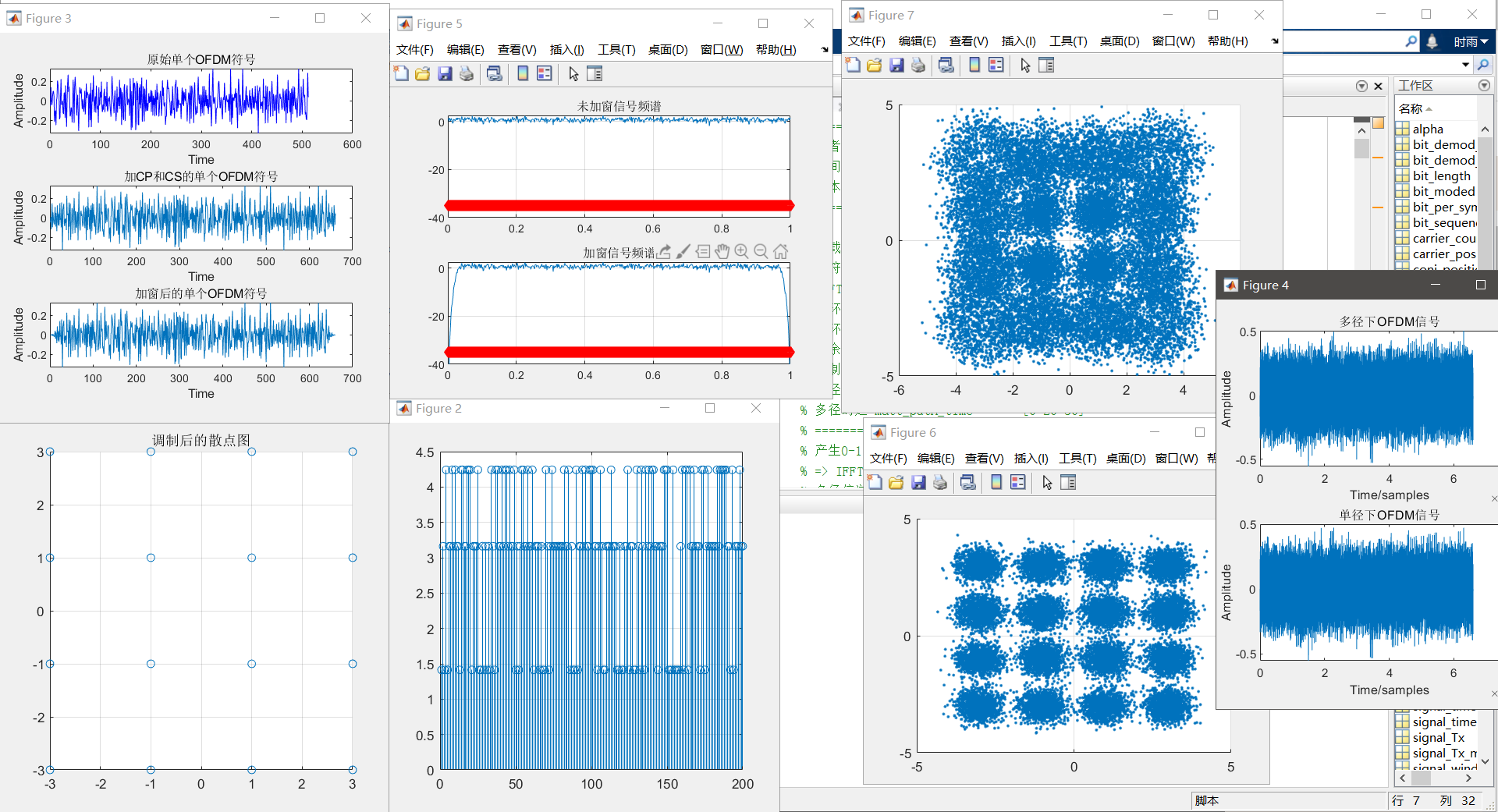自己加个循环就可以得到误码率和信噪比的曲线了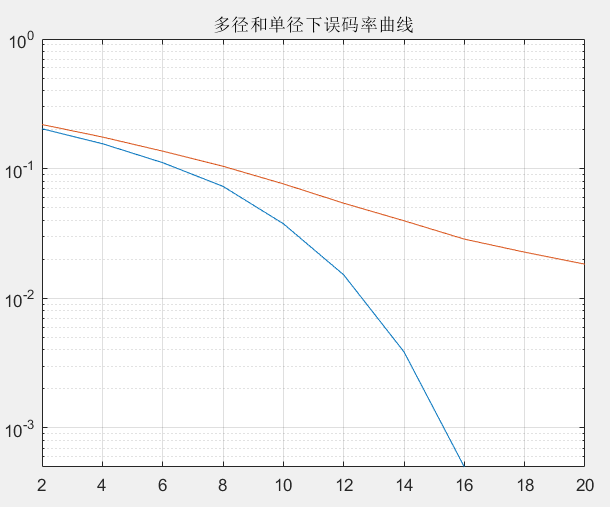展开全文matlab OFDM
• 接上篇，在对LeNet有一定理解之后，我们开始谈谈CNN里面另一个经典的模型--inception-v3 笔者在勉强读完原文之后，不得不佩服原作者对cnn研究之深入，其对cnn整体架构，参数的 调优，训练的优化，以及整个模型的...

一：背景总论

接上篇，在对LeNet有一定理解之后，我们开始谈谈CNN里面另一个经典的模型--inception-v3
笔者在勉强读完原文之后，不得不佩服原作者对cnn研究之深入，其对cnn整体架构，参数的
调优，训练的优化，以及整个模型的优化都提出了很多建设性的意见。本文将首先阐述论文的
基本思想，然后对其主要方法进行分析理解，然后再系统理解inceptionv3网络结构。前事不
忘后事之师，我们开始吧！

1. 论文背景
整个论文的思路主要是为了解决一个问题–如何处理网络更深（层数）更窄带（神经元个数/卷积核个数）来的问题，主要问题如下：
（1）：参数过多而训练数据不足的时候容易导致过拟合（如何减小参数）
（2）：当网络越来越深，带来的梯度消失，模型难以优化（如何防止梯度爆炸或梯度消失）
（3）：网络越大计算复杂度越大，难以复用（如何降低计算损失）

在论文摘要中就针对这几个问题提出了主要的解决方案–分解卷积激进正则化（这也是inception3的主要思想，待会儿会重点讲解）。
（1）：减少参数：卷积核大化小（例如5 * 5化成两个3 * 3）或者卷积核非对称化（3 * 3化成1*3和3 * 1两种）
（2）：减少计算损失：解决该缺点的根本方法是将全连接甚至一般的卷积都转化为稀疏连接。为了打破网络对称性和提高学习能力，传统的网络都使用了随机稀疏连接。但是，计算机软硬件对非均匀稀疏数据的计算效率很差，所以在AlexNet中又重新启用了全连接层，目的是为了更好地优化并行运算。现在的问题是有没有一种方法，既能保持网络结构的稀疏性，又能利用密集矩阵的高计算性能（incption 结构能解决的问题，下面详述）
（3）：如何避免梯度爆炸或梯度消失：BN层（下篇文章我们重点讲解）

2.论文提出的cnn设计原则
针对以上问题，以及解决办法如何执行，作者提出了许多在任何cnn网络中都值得广泛参考的建议，这些建议也是inception3的设计原则。
1：为了避免出现特征表示瓶颈，尤其是在网络的前面，应该在从输入到输出中尽量避免特征维度的严重压缩，特征表示维度应该尽量温和的减少。
2：高维度信息应该在网络局部进行处理，这样可以在卷积网络中增加非线性激活响应，可以使得网络训练得更快。（大化小分解卷积就是很好的例子）
3：空间聚合可以通过低纬嵌入，不会导致网络表示能力的降低。例如在进行大尺寸的卷积（如3*3）之前，我们可以在空间聚合前先对输入信息进行降维处理，如果这些信号是容易压缩的，那么降维甚至可以加快学习速度。
4：平衡好模型的宽度和深度，通过平衡网络每层滤波器的个数和网络的层数可以使得网络达到最佳效果。增加网络的宽度和深度都会提升网络的性能，但两者并行增加才能使网络性能最大化。

二： 重点–分解卷积

总论：分解卷积的主要目的是为了减少网络中的参数，主要方法有：大卷积分解成小卷积，分解为非对称卷积。

1，大卷积分解成小卷积

在cnn中一个5* 5 的卷积核 可以分解成两个3* 3的卷积核，这样可以减少28%的参数（论文作者实验所得），同时，分解后增加了一个激活函数（因为增加了一个卷积核），也就增加了非线性激活响应（也就是非线性表达能力）。使得网络的局部特征维度更加明显，加快了网络训练速度。分解示意图如下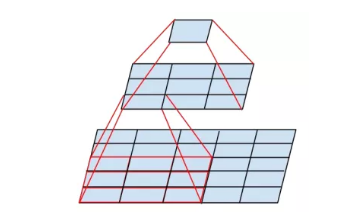结构示意图如下，简称modelA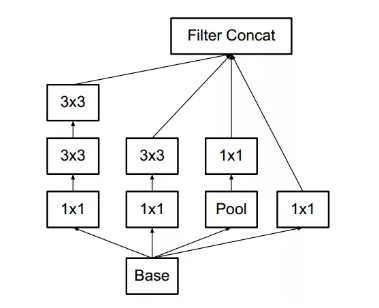到这里，各位朋友肯定在想经过分解操作之后，虽然会对局部特性的表征能力有提升，但是对于整个模型的表征性能会不会有负面作用呢？作者通过实验回答了这个问题–不会。如下图，
蓝色的虚线是通过对分解卷积使用两次Relu（都能看到这了，我就不解释什么是relu了）得到的精度–77.2%。而红色的线是对未经过分解的卷积核通过liner+relu得到的精度–76.2%。所以经过卷积核分解不会降低模型的整体表征能力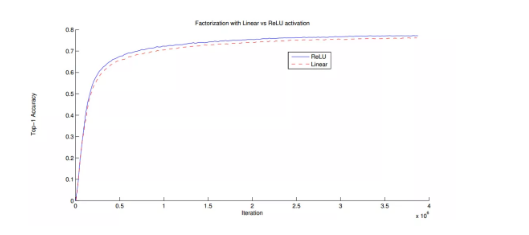2，分解为非对称卷积

例如将一个33的卷积分解成一个13卷积和一个3*1卷积，这样可以减少33%的参数，分解示意图如下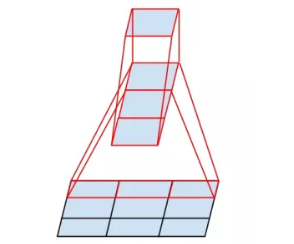结构示意图如下，简称model B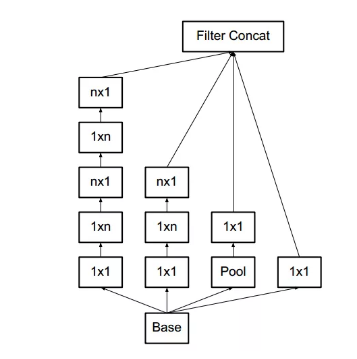其他非对称卷积如下，简称model C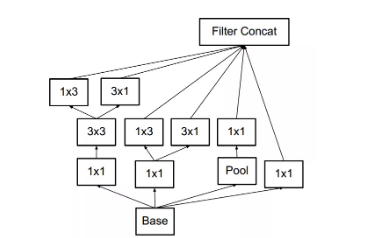3，辅助分类器

在inception-v1中，使用了辅助分类器，其主要作用是用来帮助梯度回传，加深网络的深度。inception–v3也使用了辅助分类器，但其主要作用是用作正则化器，这是因为辅助分类器做过归一化处理，或者有一个dropout层，其网络主分类器效果会更好。这也从侧面证明了归一化可以作为正则化器使用。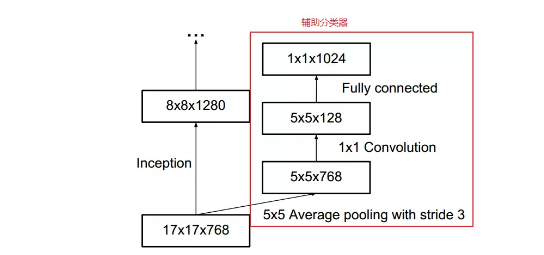4，有效的特征网格大小缩减

传统上，卷积神经网络通过池化操作来减小特征图的网格大小。为了避免特征表示瓶颈，除了作者提出的在网络的初期，避免特征维度的剧烈压缩之外，更重要的是在最大池化或者平均池化前，应增大网络滤波器激活的维数（也就是增大网络特征表征数量和能力），作者设计了一个结构，可以有效减少计算量和参数。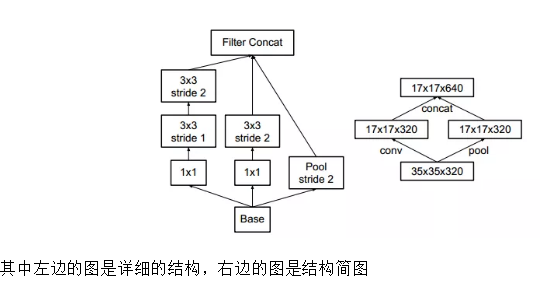三：inception和inception–v3结构

1，inception结构的作用（inception的结构和作用
作用：代替人工确定卷积层中过滤器的类型或者确定是否需要创建卷积层或者池化层。即：不需要人为决定使用什么过滤器，是否需要创建池化层，由网络自己学习决定这些参数，可以给网络添加所有可能值，将输入连接起来，网络自己学习需要它需要什么样的参数。
inception结构工作原理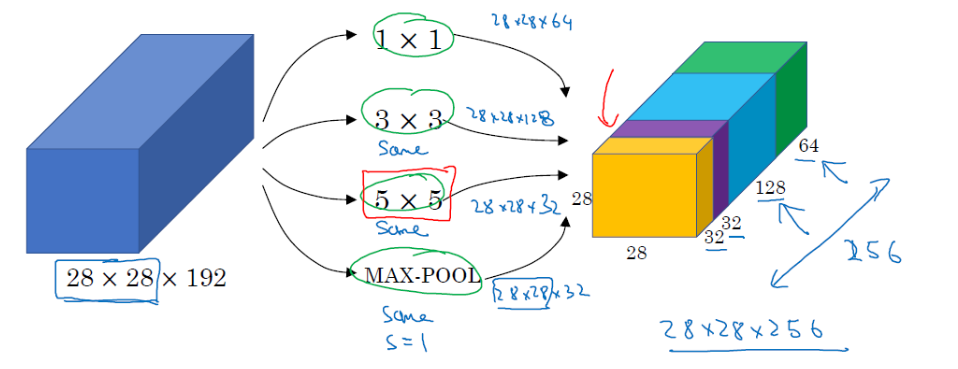2，inception结构的主要思想–用密集成分来近似最优的局部稀疏解（如上图）
（1）：采用不同大小的卷积核意味着有不同大小的感受野，最后的拼接意味着不同尺度特征的融合。
（3： 文章说很多地方都表明pooling挺有效，所以Inception里面也嵌入了pooling。
（4）：.网络越到后面特征越抽象，且每个特征涉及的感受野也更大，随着层数的增加，3x3和5x5卷积的比例也要增加。
（5）：最终版inception，加入了1x1 conv来降低feature map厚度。如果简单的将前面的结构应用到feature map上的话，concat起来的feature map厚度将会很大，所以在googlenet中为了避免这一现象提出的inception具有如下结构，在3x3前，5x5前，max pooling后分别加上了1x1的卷积核起到了降低feature map厚度的作用。（如下图）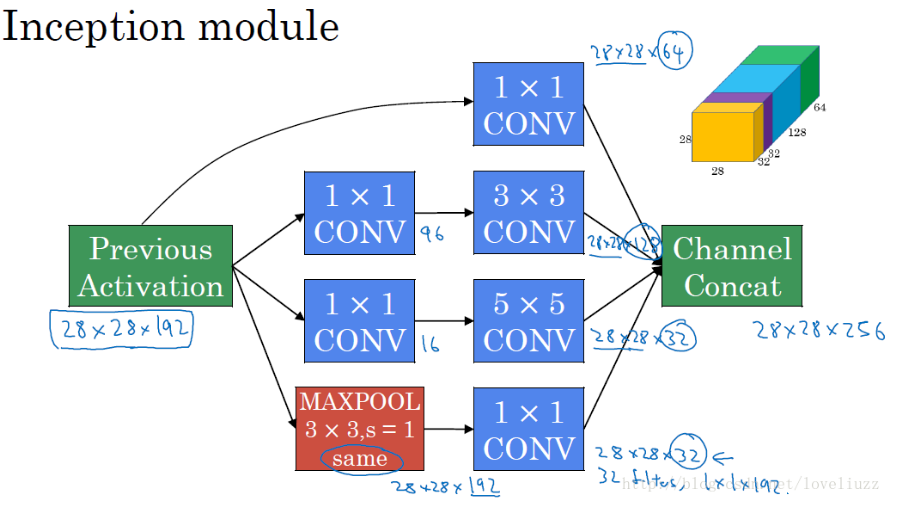inception–v3结构

Inception架构的主要思想是找出如何用密集成分来近似最优的局部稀疏结。2015 年 2 月， Inception V2 被提出， InceptionV2 在第一代的基础上将 top- 5错误率降低至 4.8% 。Inception V2 借鉴了 VGGNet 的设计思路，用两个 3 x 3 的卷积代替 5×5的大卷积。另外，在论文中，还首次提出了著名的 BN (BatchNormalization ）方法。BN是一种非常有效的正则化方法。在对神经网络的某层使用BN 方法时，每一个 mini-batch 数据的内部都会进行标准化（Normalization）处理，使输出规范化到 N C0,1 ）的正态分布。根据这篇论文中对 BN 的解释，传统的深度神经网络在训练时，尽管可以用堆砌相同结构的网络来达到提升网络性能的目的，但是这些网络层的输入都是在变化的，输入输出可能分布不一致，对特征的提取带来很大的障碍，也使得基于梯度下降的训练方式显得非常困难。在这种情况下，训练的过程只能采用一个较小的学习率。对每一层使用BN 方法则可以有效地解决这个问题，通过规范每一层的输出，使得输入输出满足同一正太分布，而其他网络层得到的输入的变化就小多了，这样，学习的效果会好很多而且比较有说服力。InceptionV3 网络是由 Google 开发的一个非常深的卷积网络。2015年 12 月， Inception V3 在论文《Rethinking the Inception Architecture forComputer Vision》中被提出，Inception V3 在 Inception V2 的基础上继续将 top-5的错误率降低至 3.5% 。Inception V3对 Inception V2 主要进行了两个方面的改进。首先，Inception V3 对 Inception Module 的结构进行了优化，现在 Inception Module有了更多的种类（有 35 × 35 、 1 7× 17 和 8× 8 三种不同结构），并且 Inception V3 还在 Inception Module 的分支中使用了分支（主要体现在 8x8 的结构中），如下图(a)，图(b)，图©。其次，在 Inception V3 中还引入了将一个较大的二维卷积拆成两个较小的一维卷积的做法。例如， 7× 7 卷积可以拆成 1×7 卷积和7 × l卷积。当然3x3 卷积也可以拆成 Ix3 卷积和 3 × l卷积。这被称为Factorizationinto small convolutions 思想。在论文中作者指出，这种非对称的卷积结构拆分在处理更多、更丰富的空间特征以及增加特征多样性等方面的效果能够比对称的卷积结构拆分更好，同时能减少计算量。例如，2个33代替1个55减少28%的计算量，如下图所示。总结
该节主要讲述了InceptionNet模型的主要特点和相比之前的神经网络改进的地方，另外讲述了BN的原理与作用，而后给出了InceptionNet-V3中减少训练计算量的方法，最后给出InceptionNet-V3的模型结构。详细的inception1-4可见该文章

展开全文• 使用数字签名技术产生的数据， 签名的对象包括了原始文件信息、 签名参数、 签名时间等信息。广泛的运用在知识产权保护、 合同签字、 金融帐务、 电子报价投标、 股票交易等方面。 这是百度百科的含义，简单来讲...

前言

电影推荐系统是数学建模培训中一次例题，网上对相同类型的模型已有答案，但相关代码跑起来仍然存在些许bug，本文基于同类型的基础上能帮助后来的学习者。

电影推荐系统即通过用户的历史观影记录，结合相同爱好用户集群，在海量的影视资源中精确对每一位用户推荐电影。本文调用R语言Recommenderlab包做电影推荐系统。

R语言 电影推荐系统

实际上，在R语言的Recommenderlab保中，对电影进行推荐，是对用户没有看过的电影做一个预测评分，通过TOP-N部电影，选取所需要的电影数量推荐表。

案例及代码

数据准备

电影数据来源于http://grouplens.org/datasets/movielens/ 网站，本文分析的数据是ml-latest-small中Ratings.csv文件，总共有100,836个评分，来自610用户对9724部电影评分。
首先安装R语言的程序包：做推荐系统的Recommenderlab包，画图的ggplot2包，数据处理的reshape包。
需要注意的是Recommenderlab针对RealRatingMatrix数据类型，总共提供了6种模型：基于项目协同过滤（IBCF）， 主成分分析（PCA）， 基于流行度推荐（POPULAR）,随机推荐（RANDOM）,奇异值分解（SVD），基于用户协同过滤算法（UBCF）。
我在这里只用了三种，感兴趣的可以将6种都比较一下，然后选取误差值最小的作为最优解。

数据预处理

调用程序包，读取数据ratings.csv，

library(recommenderlab)
library(reshape)
library(ggplot2)

数据总共4列，usersId,MoviesId,ratings还有timestamp，timestamp是一个能表示一份数据在某个特定时间之前已经存在的、 完整的、 可验证的数据,通常是一个字符序列，唯一地标识某一刻的时间。使用数字签名技术产生的数据， 签名的对象包括了原始文件信息、 签名参数、 签名时间等信息。广泛的运用在知识产权保护、 合同签字、 金融帐务、 电子报价投标、 股票交易等方面。
这是百度百科的含义，简单来讲就是把年月日的计日方式变成了十进制数据，这里提供一个网站进行转义https://tool.lu/timestamp

时间列是我们不需要的，剔除，还剩三列。

mydata<-mydata[,-4] #剔除时间戳列

看一下前6行的评分

V1 V2  V3
1  1  1 4.0
2  5  1 4.0
3  7  1 4.5
4 15  1 2.5
5 17  1 4.5
6 18  1 3.5

看一下评分(rating)的总体占比情况

prop.table(table(mydata[, 3]))
summary(mydata[, 3])

0.5          1        1.5          2        2.5          3        3.5
0.01358642 0.02787695 0.01776151 0.07488397 0.05503987 0.19880797 0.13027093
4        4.5          5
0.26595660 0.08480106 0.13101472

Min. 1st Qu.  Median    Mean 3rd Qu.    Max.
0.500   3.000   3.500   3.502   4.000   5.000

数字太多了，看看饼图

gplot(mydata,x=V3,aes(x=factor(1),fill=factor(V3)))
+geom_bar(width = 1)+coord_polar(theta="y")‘
+ggtitle("评分分布图")
+labs(x="",y="")+guides(fill=guide_legend(title = '评分分数'))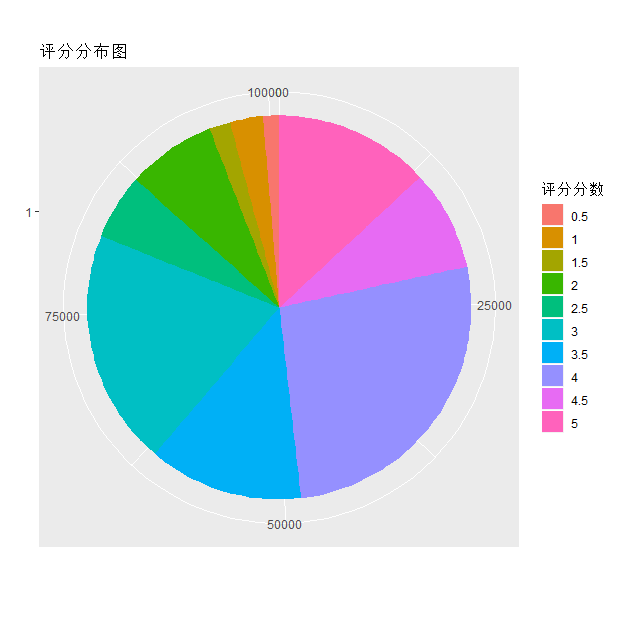图中可以看出来，打1、2分的人很少，3，4分的占了6成左右。

建立模型

调用reshape包的cast()函数将数据转换为usersId为行，moviesId为列的矩阵，删除第一列数据序号列，这个时候的数据包含两种类型:cast_df，data.frame，其中cast_df是不能直接转换为matrix的，因此需要去掉这个类属性，只保留data.frame 也就是数据框
注意转换后是一个610*9724的超大矩阵，导致数据源评分矩阵是一个非常稀疏、含有许多空缺值的矩阵但并不影响我们的后续操作。为了让数据成为Recommenderlab包能够处理的类型，转换为RealRatingMatrix。

mydata<-cast(mydata,V1~V2,value="V3",fun.aggregate=mean) #生成一个以v1为行，v2为列的矩阵，使用v3进行填充
mydata<-mydata[,-1] #第一列数字为序列，可以删除
class(mydata)
class(mydata)<-"data.frame"  #只选取data.frame
mydata<-as.matrix(mydata)
mydata<-as(mydata,"realRatingMatrix")
mydata

给生成的矩阵的列命名"M_moviesId"表示电影编号，调用Recommenderlab的UBCF（基于用户的协同过滤算法）模型
补个知识点：协同过滤主要分为两个步骤，首先依据目标用户的已知电影评分找到与目标用户观影风格相似的用户群，然后计算该用户群对其他电影的评分，并作为目标用户的预测评分，协同过滤算法的具体细节请参考文献。

colnames(mydata)<-paste0("M",1:9726,sep="")
mydata.model <- Recommender( mydata[1:610], method = "UBCF")

数据处理完毕，接来下是进行预测，以编号为233的用户为例，预测他对前6部电影的评分（看过的电影不会再预测，显示为NA）

mydata.predict <- predict(mydata.model,mydata, type="ratings")
as(mydata.predict,"matrix")[1,1：6]

M1       M2       M3       M4       M5       M6
NA 3.282566 3.263601 3.308725 3.332142 3.308725

现在你可以命令R给编号为233的用户推荐5部预测评分高的电影表

mydata.predict2 <- predict( mydata.model,  mydata, n=5) #n指推荐电影数量
as(mydata.predict2,"list")

$233  "M1067" "M969" "M1218" "M977" "M2611" 到这里整个推荐系统模型已经建立好了。 展开全文R语言 推荐系统 • LDPC各类译码方法的MATLAB实现主要内容新的改变功能快捷键合理的创建标题，有助于目录的生成如何改变文本的样式插入链接与图片如何插入一段漂亮的代码片生成一个适合你的列表创建一个表格设定内容居中、居左、居右... LDPC译码：和积译码算法（SPA）、最小和算法（MSA）、分层译码算法（LBP）、动态信息更新策略IDS（含RBP、NW-RBP、SVNF-RBP）的MATLAB实现 主要内容 LDPC码是一种接近香农极限的“好”码，自二十世纪九十年代被重发现以来，已获得越来越广泛的应用，成为诸多通信领域推荐使用的信道编码。近来，3GPP将其作为5G新空口eMMB应用上的数据传输编码方案。本文将主要介绍LDPC的一些主要译码方法并通过MATLAB实现，这里主要使用的译码方法为SPA（ Sum-Product Algorithm和积译码算法）、LBP（ Layered-Belief Propagation分层置信传播译码算法）以及性能更高的IDS(Information Dynamic Schdule)类译码算法如RBP（ Residual Belief Propagation）、NW-RBP（ Node Wise-RBP）、SVNF-RBP（ Silent-Variable-Node-Free RBP）。 符号说明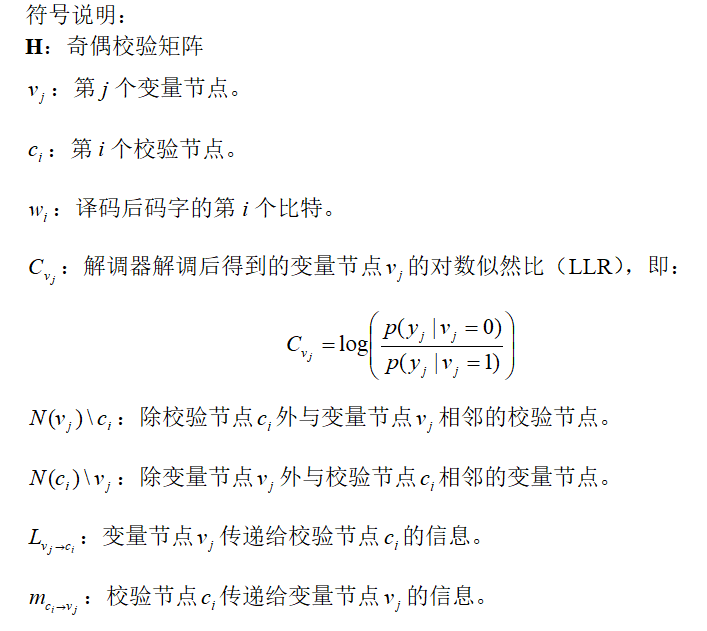Check to Variable: C2V Variable to Check: V2C 算法说明与代码实现 和积译码算法（SPA） 基本原理 LDPC码的概率域BP算法的变量节点和校验节点操作有大量乘法，这样计算量和复杂度都很高，如果将上述０和１的概率信息用对数似然比表示，就可以把乘法运算转换为加法运算，大大降低了运算量，此算法称为和积译码算法。步骤如下： 1. 初始化 L v j → c i = C v j L_{v_{j} \rightarrow c_{i}}=C_{v_{j}} （1） 2. 校验节点更新 m c i → v j = 2 × atanh ⁡ ( ∏ v b ∈ N ( c i ) \ v j tanh ⁡ ( L v b → c i 2 ) ) m_{c_{i} \rightarrow v_{j}}=2 \times \operatorname{atanh}\left(\prod_{v_{b} \in N\left(c_{i}\right) \backslash v_{j}} \tanh \left(\frac{L_{v_{b} \rightarrow c_{i}}}{2}\right)\right) （2） 3. 变量节点更新 L v j → c i = ∑ c a ∈ N ( v j ) \ c i m c a → v j + C v j L_{v_{j} \rightarrow c_{i}}=\sum_{c_{a} \in N\left(v_{j}\right)\backslash c_{i}} m_{c_{a} \rightarrow v_{j}}+C_{v_{j}} （3） 4. 后验概率信息更新 L j = ∑ c a ∈ N ( v j ) m c a → v j + C v j L_{j}=\sum_{c_{a} \in N\left(v_{j}\right)} m_{c_{a} \rightarrow v_{j}}+C_{v_{j}} （4） MATLAB代码实现SPA function Dec_out = LdpcDecode_SPA(in,H,MaxIter) %%% in为解调后的的对数似然比信息，为N维行向量 %%% H为校验矩阵,MaxIter为最大迭代次数 [M,N] = size(H); %% 初始化 V_n = in; %似然概率 V_mn = repmat(V_n,M,1); %m代表校验节点索引，n代表变量节点索引 V_mn(H == 0) = 0; U_mn = zeros(M,N); %% 译码 for i = 1:MaxIter % 校验节点更新 for m = 1:M Nm = find(H(m,:)==1); %校验节点m相邻的变量节点 for n = 1:length(Nm) aa = Nm; aa(n) = []; % MSA: U_mn(m,Nm(n)) = prod(sign(V_mn(m,aa)))*min(abs(V_mn(m,aa))); U_mn(m,Nm(n)) = 2*atanh(prod(tanh(V_mn(m,aa)/2))); end end %变量节点更新 for n = 1:N Mn = find(H(:,n)==1); %变量节点n相邻的校验节点 for m = 1:length(Mn) bb = Mn; bb(m) = []; V_mn(Mn(m),n) = in(n) + sum(U_mn(bb,n)); end V_n(n) = in(n) + sum(U_mn(Mn,n));%似然概率更新 end end %% 硬判决 decBits = zeros(1,N); decBits(V_n <= 0) = 1; %信息位+校验位的译码输出 Dec_out = decBits(1:N-M); %仅含信息位的译码输出 注意最小和算法（MSA）与SPA的区别仅在于校验节点更新，MSA算法利用tanh、atanh的单调性，将其非线性运算转化为最招序列中最小值而减少计算量，将MSA的校验节点更新公式为: m c i , v j = min ⁡ v s ∈ N ( c i ) v j ( L v b → c i ∣ ) × ∏ v b ∈ N ( c i ) v j sgn ⁡ ( L v b − c i ) m_{c_{i}, v_{j}}=\min _{v_{s} \in N\left(c_{i}\right) v_{j}}\left(L_{v_{b} \rightarrow c_{i}} |\right) \times \prod_{v_{b} \in N\left(c_{i}\right) v_{j}} \operatorname{sgn}\left(L_{v_{b}-c_{i}}\right) （5） 已在代码中作出注释，需要时进行替换即可。 分层译码算法（LBP） 基本原理 在SPA中本次的更新值仅可以在下次迭代中使用，LBP采用分层译码的方法，即将H按照水平方向分为数个子层，每层可单独进行SPA译码，上层译码更新后的信息在下层译码时即可使用，其收敛速度是SPA算法的两倍。这里我们将每个校验节点设为一个单独的层。在每一层中： 变量节点更新： L v j → c i = c v j − m c i → v j L_{v_{j} \rightarrow c_{i}}=c_{v_{j}}-m_{c_{i} \rightarrow v_{j}} 校验节点更新： m c i → v j = ∏ v b ∈ N ( c i ) \ v j sign ⁡ ( L v b → c i ) × [ ∑ v b ∈ N ( c i ) \ v j − ln ⁡ tanh ⁡ ( ∣ L v b → c i ∣ 2 ) ] m_{c_{i} \rightarrow v_{j}}=\prod_{v_{b} \in {N}\left(c_{i}\right) \backslash v_{j}} \operatorname{sign}\left(L_{v_{b} \rightarrow c_{i}}\right) \times\left[\sum_{v_{b} \in N\left(c_{i}\right) \backslash v_{j}}-\ln \tanh \left(\frac{\left|L_{v_{b} \rightarrow c_{i}}\right|}{2}\right)\right] 后验概率更新则变为： L j = L v j → c i + m c i → v j L_{j}=L_{v_{j} \rightarrow c_{i}}+m_{c_{i} \rightarrow v_{j}} 硬判决方法不变 MATLAB代码实现LBP https://github.com/vodafone-chair/5g-nr-ldpc/blob/master/ldpcDecode.m 注意这里的代码经过了少许修改 function Dec_out = LdpcDecode_LBP(in,H,MaxIter) [M,N] = size(H); minVal = realmin('double'); numEntries = nnz(H); Rcv = spalloc(M, N, numEntries); Qv = in; % Decode for ldpcCurIter = 1:MaxIter % Loop over all check nodes for checkIdx = 1:M % Find all neighbouring variable nodes of current check node nbVarNodes = find(H(checkIdx,:)==1); % Tmp update llr tmpLlr = Qv(nbVarNodes) - full(Rcv(checkIdx,nbVarNodes)); % Compute S = (Smag, Ssign) Smag = sum(-log(minVal+tanh(abs(tmpLlr)/2))); % Count number of negative elements if mod(sum(tmpLlr<0),2) == 0 Ssign = +1; else Ssign = -1; end % Loop all neighbouring variable nodes for varIter = 1:length(nbVarNodes) varIdx = nbVarNodes(varIter); Qtmp = Qv(varIdx) - Rcv(checkIdx, varIdx); QtmpMag = -log(minVal+tanh(abs(Qtmp)/2)); % Note: +minVal in order to deal with llr=0; % implementation can be improved QtmpSign = sign(Qtmp+minVal); % Update message passing matrix % From reference: Rcv = phi^-1(S-phi(Qtmp)) Rcv(checkIdx, varIdx) = Ssign*QtmpSign * (-log(minVal+tanh(abs(Smag-QtmpMag)/2))); % Update Qv. From reference: Qv = Qtmp + Rcv Qv(varIdx) = Qtmp + Rcv(checkIdx, varIdx); end end Dec_out = zeros(1,N-M); Dec_out(Qv(1:N-M)<0) = 1; end 代码中的译码顺序是从H矩阵的第一行顺序译码，事实上可以根据码的结构调整译码顺序，合理的安排译码顺序会提高误码性能。 IDS类算法 在SPA或者LBP的迭代过程中，一些节点产生的信息可能在少量迭代时即已趋于稳定，而另一部分信息可能徐国更多次的迭代才能稳定下来，而SPA或LBP并不对节点进行区分。IDS类方法考虑节点（变量节点或校验节点）信息的变化，有选择的挑选Tanner图中的边进行信息的更新，实验证明，这类方法在收敛速度与误码性能上较前两种方法更高。 后面介绍的几种IDS类方法均是基于贪婪的准则选择相应的边进行更新，考虑的均为校验节点处的信息变化。 单次迭代的终止条件及判决方法 假设Tanner图中边的总数为E，即校验矩阵H中非零元素数量。通过分析可以发现SPA、LBP的C2V更新次数均为E，下面介绍的IDS类方法考虑的均为校验节点信息的变化，因此当校验节点的更新次数（注意不包括计算残差时的校验节点更新）达到E时本次迭代结束。 其后验概率更新、硬判决方式与SPA相同，不再赘述。 残差置信传播（RBP） 算法内容 RBP是每次选择校验节点中信息变化最大的边进行更新，考察信息量变化的准则是相邻两次校验节点更新的值得变化量，称为残差，即： r c i → v j = ∣ m c i → v j p r e − m c i → v j ∣ r_{c_{i} \rightarrow v_{j}}=\left|m_{c_{i} \rightarrow v_{j}}^{p r e}-m_{c_{i} \rightarrow v_{j}}\right| （6） 可以看到计算残差即是做校验节点更新，然后取与上一次校验节点更新的变化量。但获得残差仅仅是为了进行比较得到具有最大残差的边，而并不进行传播，如上所述，采用SPA更新校验节点的计算量比较大，因此我们使用（5）式即MSA采用的校验节点更新方法计算残差，进行传播时再采用（2）式计算精确的校验节点更新值。实验证明，这种方式对实验结果影响很小，却大幅度较少了计算量。下面介绍的另外几种IDS方法也采取同样的残差计算策略。 在每次迭代中首先选取具有最有最大残差值的边，假设该边是 c i → v j c_{i} \rightarrow v_{j} ，使用（2）式计算 m c i → v j m_{c_{i} \rightarrow v_{j}} 并置 r c i → v j = 0 r_{c_{i} \rightarrow v_{j}}=0 ；之后使用（3）式计算变量节点更新 v j → c a v_{j} \rightarrow c_{a} 其中 c a ∈ N ( v j ) \ c i c_{a} \in N\left(v_{j}\right) \backslash c_{i} ；最后使用式（6）更新残差值 r c a → v b r_{c_{a}} \rightarrow v_{b} ，其中 v b ∈ N ( c a ) \ v j v_{b} \in N\left(c_{a}\right) \backslash v_{j} 。判断终止条件是否达到，否则继续执行上述过程。该过程可整理如下：下面举例说明更新过程（本文中用红色线条表示执行校验节点更新的边，蓝色线条表示变量节点更新的边，黄色线条表示残差更新的边），一码字的Tanner图如下图所示： 1. 假设经计算选择了边 C 4 → V 3 \mathcal{C}_{4} \rightarrow \mathcal{V}_{3}更新 m c 4 → v 3 m_{c_{4} \rightarrow v_{3}} 并置 r c 4 → v 3 = 0 r_{c_{4} \rightarrow v_{3}}=0 2. 根据(3)式更新 v 3 → c 2 \mathcal{v}_{3} \rightarrow \mathcal{c}_{2} v 3 → c 3 \mathcal{v}_{3} \rightarrow \mathcal{c}_{3}3. 根据(5)(6)式更新 r c 2 → v 2 r_{c_{2} \rightarrow v_{2}} r c 3 → v 4 r_{c_{3} \rightarrow v_{4}} r c 3 → v 5 r_{c_{3} \rightarrow v_{5}}RBP的贪婪性 RBP的策略即是优先更新低可靠性的信息用以“纠正”变量节点，这在直观上是可行的，但由于其选择边的贪婪特性，当图中存在“贪婪组”时，最大残差边的选取很可能在一个该贪婪组中重复选择，这样虽然校验节点或变量节点的信息在不断更新，但却没有新的信息加入，这样当迭代次数增大时并不能提升译码性能。仍采用上面的例子来进一步解释贪婪组：该图a中用粗实线表示表示贪婪组中的边共7条，贪婪组中边的总数仅占总边数的一小部分。最大残差边的选取在该贪婪组中以一种固定的顺序被选取,该顺序如图b所示。在该贪婪组中，最大残差值虽然在不断变化，但该贪婪组中却并没有新的信息加入，信息的不完整性注定其不能达到最优的性能，而RBP却在其中浪费了大量的计算资源。实验结果表明，由于对产生信息的节点进行的选择，RBP有着更快的收敛速度，但由于贪婪性，其十分容易陷入贪婪组中，因而相比于SPA、LBP有着更高的误码平层（error floor）。由于SPA、LBP对节点不进行选取，不具贪婪性，因此这些方法不会产生贪婪组。 下面介绍的NW-RBP与SVNF-RBP是针对于RBP算法的改进版，尽量避免信息的更新陷入贪婪组。 NW-RBP (Node wise RBP) 为避免陷入贪婪组中，可以选择多条边进行信息更新。在每次迭代中首先选取具有最有最大残差值的边，假设该边是 c i → v j c_{i} \rightarrow v_{j} ，使用（2）式计算 m c i → v k m_{c_{i} \rightarrow v_{k}} ,其中 v k ∈ N ( c i ) v_{k} \in N\left(c_{i}\right) 并置 r c i → v k = 0 r_{c_{i} \rightarrow v_{k}}=0 （注意这里与RBP的区别，RBP是选择 c i → v j c_{i} \rightarrow v_{j} 一条边，而NW-RBP是选择校验节点 c i c_{i} 连接的所有边）；之后做变量节点更新 L v k → c a L_{v_{k} \rightarrow c_{a}} ，其中 c a ∈ N ( v k ) \ c i c_{a} \in N\left(v_{k}\right) \backslash c_{i} ；最后更新 r c a → v b r_{c_{a} \rightarrow v_{b}} ，其中 v b ∈ N ( c a ) \ v k v_{b} \in N\left(c_{a}\right) \backslash v_{k} 。判断终止条件是否达到，否则继续执行上述过程。该过程可整理如下：下面举例说明边的选取过程： 1. 假设经计算选择了边 C 4 → V 3 \mathcal{C}_{4} \rightarrow \mathcal{V}_{3} ，则用(2)式计算 m c i → v k m_{c_{i} \rightarrow v_{k}} ,其中 v k ∈ N ( c i ) v_{k} \in N\left(c_{i}\right)2. 使用（3）式计算 L v k → c a L_{v_{k} \rightarrow c_{a}} ，其中 c a ∈ N ( v k ) \ c i c_{a} \in N\left(v_{k}\right) \backslash c_{i}3. 使用(5)(6)计算 r c a → v b r_{c_{a} \rightarrow v_{b}} ，其中 v b ∈ N ( c a ) \ v k v_{b} \in N\left(c_{a}\right) \backslash v_{k}这样通过选取更多的边就可以降低进入贪婪组的概率。 SVNF-RBP (Silent-Variable-Node-Free RBP) RBP通过更新更多的边来来避免陷入贪婪组，但这些边携带的信息可能对译码提升的性能贡献可能很小。另外在迭代过程中我们发现，一些变量节点可能永远没有机会得到更新，这样的变量节点称为Silent Variable Nodes，这些节点的存在会影响译码性能。不同于NW-RBP选取最大残差边对应的校验节点连接的所有边进行校验节点更新，SVNF-RBP让每一个变量节点都有同样的机会去选取其连接的具有最大残差的边，这样就保证了所有被选取的边所携带的信息尽可能的大，同时消除了Silent Variable Nodes。且由于其选取的校验节点更新的边更多，故会进一步降低陷入贪婪组中的危险。 其执行过程如图所示：这里不再画图示范，选取具有最大残差边后的更新过程与RBP算法完全相同。与RBP的区别仅在于，RBP选取的最大残差边拥有全局最大的残值，而SVNF-RBP选取的边仅是该边对应的变量节点所连接的边中，其残差最大。 注意到4~13行执行次数达到 d c ‾ \overline{d_{c}} （该LDPC码的平均重量）次时，校验节点更新次数为E次，此时一次迭代完成。 MATLAB代码实现 function Dec_out = LdpcDecode_IDS(in,H,MaxIter,Method) % The input "in" is a 1*N vector which contains the log-likehood ratio that % calculated by demodulator. H is the parity check matrix of the LDPC % codes. MaxIter defines the maximun iteration of the decoder. The decode % algorith controlled by parameter "Method", the choices are 'RBP','NW-RBP', % 'SVNF-RBP' % Reference: "Informed Dynamic Scheduling for Belief-Propagation Decoding % of LDPC Codes" By Andres. 'RBP','NW_RBP' % "Two Informed Dynamic Scheduling Strategies for Iterative LDPC Decoders" % By Huang-Chang Lee. 'SVNF_RBP' % Author: Wang Bingbing % Last Update: 2019/10/17 %% Initialization [M,N] = size(H); V_mn = repmat(in,M,1); V_mn(H == 0) = 0; U_mn = zeros(M,N); ResC2V = zeros(M,N); % Initialize the residual martix. for m = 1:M Nm = find(H(m,:)==1); % We use "Nm" to represent a check node's neighbour variable nodes. for n = 1:length(Nm) aa = Nm; aa(n) =[]; ResC2V(m,Nm(n)) = prod(sign(V_mn(m,aa)))*min(abs(V_mn(m,aa))); end end %% Decode % Note that one iteration will be finished after the number of C2V % message updates equal to the number of edges in LDPC graph switch Method case 'RBP' cntCVmax = nnz(H); for j = 1:MaxIter cntCV = 0; while cntCV ~= cntCVmax % Choose Ci to Vj [~,IndMax] = max(abs(ResC2V(:))); [ci,vj] = ind2sub(size(ResC2V),IndMax); % Generate and propagate Ci to Vj and then % set r(ci,vj)=0 Nm_ci = find(H(ci,:)==1); Nm_ci(Nm_ci == vj) = []; U_mn(ci,vj) = 2*atanh(prod(tanh(V_mn(ci,Nm_ci)/2))); ResC2V(ci,vj) = 0; cntCV = cntCV+1; % Update information Vj to its neighbour check % nodes except Ci Mn_vj = find(H(:,vj)==1); for c = 1:length(Mn_vj) ca = Mn_vj(c); if ca == ci continue end bb = Mn_vj; bb(c) = []; V_mn(ca,vj) = in(vj) + sum(U_mn(bb,vj)); % Update residual from ca to its neighbour % variable nodes ecxept vj Nm_ca = find(H(ca,:)==1); for v = 1:length(Nm_ca) vb = Nm_ca(v); if vb == vj continue end cc = Nm_ca; cc(v) =[]; ResC2V(ca,vb) = prod(sign(V_mn(ca,cc)))*min(abs(V_mn(ca,cc)))-ResC2V(ca,vb); end end end end case 'SVNF-RBP' averDv = sum(sum(H))/N; % Average of variable nodes' degree for j = 1:MaxIter for loopInd = 1:averDv for vj = 1:N % find (Cmax,Vmax) Mn = find(H(:,vj) == 1); ResC2V_tmp = ResC2V(Mn,:); ResC2V_tmp(:,vj) = 0; [~,IndMax] = max(abs(ResC2V_tmp(:))); [mt, Vmax] = ind2sub(size(ResC2V_tmp),IndMax); Cmax = Mn(mt); % Generate information Cmax to Vmax and propagate, % then set r(Cmax,Vmax)=0 Nm_Cmax = find(H(Cmax,:) == 1); Nm_Cmax(Nm_Cmax == Vmax) = []; U_mn(Cmax,Vmax) = 2*atanh(prod(tanh(V_mn(Cmax,Nm_Cmax)/2))); ResC2V(Cmax,Vmax) = 0; % Update information Vmax to its neighbour check % nodes except Cmax Mn_Vmax = find(H(:,Vmax) == 1); for c1 = 1:length(Mn_Vmax) Ca = Mn_Vmax(c1); if Ca == Cmax continue end bb = Mn_Vmax; bb(c1) = []; V_mn(Ca,Vmax) = in(Vmax) + sum(U_mn(bb,Vmax)); % Update residual from Ca to its neighbour % variable nodes ecxept Vmax Nm_Ca = find(H(Ca,:) == 1); for v1 = 1:length(Nm_Ca) Vb = Nm_Ca(v1); if Vb == Vmax continue end cc = Nm_Ca; cc(v1) =[]; % For reducing complexity, using MS to % calculate redidual and using SPA to % propagate. ResC2V(Ca,Vb) = prod(sign(V_mn(Ca,cc)))*min(abs(V_mn(Ca,cc)))-ResC2V(Ca,Vb); end end end end end case 'NW-RBP' for j = 1:MaxIter for i = 1:M % Choose Ci to Vj [~,IndMax] = max(abs(ResC2V(:))); [ci,~] = ind2sub(size(ResC2V),IndMax); % Generate and propagate ci to its neighbour check nodes Nm_ci = find(H(ci,:) == 1); for v1 = 1:length(Nm_ci) vk = Nm_ci(v1); aa = Nm_ci(Nm_ci ~= vk); U_mn(ci,vk) = 2*atanh(prod(tanh(V_mn(ci,aa)/2))); ResC2V(ci,vk) = 0; % Generate and propagate vk to its neighbour check % nodes except ci Mn_vk = find(H(:,vk) == 1); for c1 = 1:length(Mn_vk) ca = Mn_vk(c1); if ca == ci continue end bb = Mn_vk(Mn_vk ~= ca); V_mn(ca,vk) = in(vk) + sum(U_mn(bb,vk)); % Compute residual ca to its neighbour variable % nodes except vk Nm_ca = find(H(ca,:) == 1); for v2 = 1:length(Nm_ca) vb = Nm_ca(v2); if vb == vk continue end cc = Nm_ca(Nm_ca ~= vb); ResC2V(ca,vb) = prod(sign(V_mn(ca,cc)))*min(abs(V_mn(ca,cc)))-ResC2V(ca,vb); end end end end end end V_n = in + sum(U_mn); decBits = zeros(1,N); decBits(V_n <= 0) = 1; % Comnination of information bits and check bits Dec_out = decBits(1:N-M); % Only information bits end 代码使用说明 本文代码的输入参数主要为三个，即in（解调器解调后得到的对数似然信息比），H（LDPC的校验矩阵）与MaxIter（译码器的最大迭代次数），使用时直接调用即可。必须注意的是参量H是完整的校验矩阵H，而非稀疏矩阵，直接采用稀疏性的参数可以大大降低内存使用量，很容易将代码转换为稀疏性的索引，读者可以自行尝试。 以上程序经验证可以正常运行，虽经过仔细检查核对，但仍不免有所疏漏，请读者在使用时务必留心验证，如发现疏漏之处请立即留言，共同探讨学习。 此外，十分重要的一点是以上代码仅供理解算法学习使用，运行效率其实很低（我在跑仿真时用的其实是C语言，但其要针对不同码字进行特定的调参，并不适合初学者，需要学习的可以去Github上搜索下载，资源很多），大家在掌握算法思想后可以自行探索如何让优化。 参考文献  R. G. Gallager, Low-Density Parity-Check Codes. Cambridge, MA: M.I.T. Press, 1963.  D. J. C. MacKay, “Good error-correcting codes based on very sparse matrices,” IEEE Trans. Inform. Theory, vol. 45, pp. 399–431, Mar. 1999.  D. E. Hocevar. “A reduced complexity decoder architecture via layered decoding of LDPC code” in Proc. IEEE Workshop Signal processing and Systems (SIPS. 04), Austin, TX, Oct. 2004, pp. 107-112.  Casado A I V, Griot M, Wesel R D. Informed Dynamic Scheduling for Belief-Propagation Decoding of LDPC Codes[J]. 2007.  Lee H C , Ueng Y L , Yeh S M , et al. Two Informed Dynamic Scheduling Strategies for Iterative LDPC Decoders[J]. IEEE Transactions on Communications, 2013, 61(3):886-896. 展开全文LDPC译码 SPA LBP RBP • 部分参数解析如下： ip_forward - BOOLEAN 0 - disabled (default) not 0 - enabled Forward Packets between interfaces. This variable is special, its change resets all configuration parameters to their ...网络 sysctl ipv4 network • 最近一段时间在荔枝派zero上调试simplefb进行显示视频和图片，经过一段时间的研究，基本上搞清楚了simplefb参数的设置、uboot和kernel之间关于参数的传递流程，在这里记录一下，以备查阅。 首先描述一下simplefb使...linux 嵌入式 内核 • 本博文中的代码，实现的是加载训练好的模型model_halcon_resenet.h5，并把该模型的参数赋值给两个不同的新的model。 函数式模型 官网上给出的调用一个训练好模型，并输出任意层的feature。 model = Model(inputs=... • Pytorch猫狗大战系列： 猫狗大战1-训练和测试自己的数据集 猫狗大战2-AlexNet 猫狗大战3-MobileNet_V1&V2 猫狗大战3-MobileNet_V3 TensorFlow 2.0猫狗大战系列 猫狗大战1、制作与读取record数据 猫狗大战2、训练与...pytorch 深度学习 人工智能 • Etcd的启动配置参数详解 万次阅读 2018-05-07 15:32:06 1. Etcd配置参数 1.1. member flags 1.2. clustering flags 1.3. proxy flags 1.4. security flags 1.5. logging flags 1.6. unsafe flags 1.7. profiling flags 1.8. auth flags 1.9. experimental flags ...etcd • The kernel’s command-line parameters The following is a consolidated list of the kernel parameters as implemented by the __setup(), early_param(), core_param() and module_param() macros and sorted ... • Android Camera HAL V3 Vendor Tag及V1，V3参数转换 千次阅读 热门讨论 2015-01-17 16:58:38 但是到了HAL V3，从framework到hal的参数传递都是通过metadata的方式来传递，简单的说就是每一个设置现在都变成了一个参数对，比如假设要设置AE mode为auto，以前V1可能是“AE mode=auto”这样的字符串，在V3就是... • Yolov3参数理解 万次阅读 多人点赞 2018-08-07 15:03:57 max_batches = 20200 训练达到max_batches后停止学习 policy=steps 这个是学习率调整的策略，有policy：constant, steps, exp, poly, step, sig, RANDOM，constant等方式 参考... • 但是出现错误，如ORA-01428：参数超出范围。create or replace FUNCTION Country_TOP_500(ISO_COUNTRY_CODE IN VARCHAR, For_DATE DATE)RETURN Country_TOP_500_TABLE_TYPE AS--To create a seperate transcion ... • There is also CONFIG_LOG_CPU_MAX_BUF_SHIFT config parameter that allows to increase the default size depending on the number of CPUs. See init/Kconfig for more details. logo.nologo [FB] Disables ... • 目标检测 YOLO v3 训练 人脸检测模型 万次阅读 热门讨论 2018-07-05 11:28:15 YOLO v3已经提供 COCO（Common Objects in Context）数据集的模型参数。我们可以把COCO的模型参数作为预训练参数，再结合已有的数据集，创建自己的检测算法。 本例使用WIDER FACE人脸数据，训练一个高...YOLO 人脸 • 内核引导参数大体上可以分为两类：一类与设备无关、另一类与设备有关。与设备有关的引导参数多如牛毛，需要你自己阅读内核中的相应驱动程序源码以获取其能够接受的引导参数。比如，如果你想知道可以向 AHA1542 SCSI ... • @Query、@QueryMap用于Http Get请求传递参数.如:@GET("group/users")Call> groupList(@Query("id...id=groupId")即将@Query的key-value添加到url后面组成get方式的参数,@QueryMap同理@Field用于Post方式传递参数,... • tensorflow实现inception V3 万次阅读 2018-06-26 22:58:03 本文主要通过tensorflow的contrib.slim模块来实现Google Inception V3来减少设计Inception V3的代码量，使用contrib.slim模块便可以通过少量的代码来构建有42层深的Inception V3。一、Inception V3网络结构介绍... • 使用自己的数据集训练GoogLenet InceptionNet V1 V2 V3模型（TensorFlow） 【尊重原创，转载请注明出处】https://blog.csdn.net/guyuealian/article/details/81560537 新增博客《使用自己的数据集训练MobileNet、... • yoloV3参数理解及注释 万次阅读 多人点赞 2018-07-04 09:10:26 batch=64 一批训练样本的样本数量，每batch个样本更新一次参数 subdivisions=64 batch/subdivisions作为一次性送入训练器的样本数量 如果内存不够大，将batch分割为subdivisions个子bat... • Python 中的 max/min函数是用来获取传入参数的最大值或最小值的，其运行机制都一样，所以这里主要描述 max 的运行机制以及使用方法。 最简单的用法，就是直接传入两个要对比的元素，可以返回其两个元素中的最大值。...Python max • YOLO，是You Only Look Once的缩写，一种基于深度卷积神经网络的物体检测算法，YOLO v3是YOLO的第3个版本，检测算法更快更准。 本文源码：https://github.com/SpikeKing/keras-yolo3-detection 欢迎Follow我的...Yolo keras • 模板参数自动推导 千次阅读 2014-07-11 12:54:19 就像图1所示，template中的参数为模板形参，get_max_type()中的参数为模板函数形参。当我们调用这个模板函数的时候，char,int为模板实参，'a',10为模板函数实参。 从图1的代码中，我们可以看到模板的形参和模板... • DeepLab v3 模型 2021-01-14 18:55:40 DeepLab V3模型中使用了bottleneck结构，在deeplab v3中对该结构做了调整 使用pytorch下载预训练模型 在pytorch中下载的模型与官方模型存在一点点差别， keras实现Deeplab v3 与论文中的模型存在一点差别， from ... • 先看程序实现效果 1. 设置环境 IDE：PyCharm Python：3.8 新建 project： Yolov3 安装依赖 Package, (路径: ...2. 下载对象名字coco.names, 参数文件cfg，和权重文件 weights coco.names 下载 https://github.com/pjrCNN卷积神经网络 yolo v3 yolo opencv python • 1. 保存与加载模型参数import tensorflow as tftensorflow的保存和加载是通过tf.train.Saver类实现的, 她的构造函数是def __init__(self, var_list=None, reshape=False, sharded=False, ...tensorflow 模型保存 预训练 • MobileNetV3 一种轻量级网络，它的参数量还是一如既往的小 第一列Input代表mobilenetV3每个特征层的shape变化； 第二列Operator代表每次特征层即将经历的block结构，我们可以看到在MobileNetV3中，特征提取经过了...卷积 深度学习 卷积神经网络 网络 神经网络 • 这两天在使用yolov3-tiny，记录下一些训练参数和其取值的意义。 在不检测目标占比小的情况时，可以选用的yolov3-tiny模型 1.模型训练参数 yolo训练时输出的各项参数（这图用的是yolov3-tiny训练，所以只有16和23这... • zero+ pjw@pjw-virtual-machine:~/Allwinner/V3S/linux$ 五、烧录 准备： boot.scr(主线llinux)，参考：u-boot传参(boot.scr)和参数配置(script.bin)文件 设备树sun8i-v3s-licheepi-zero.dtb或者sun8i-v3s-licheepi-...
• 我正在尝试使用PyTorchViz...forward_hooks.values(): 491 hook_result = hook(self, input, result) TypeError：forward（）缺少1个必需的位置参数：“ hidden” 我该怎么做才能解决这个问题？ 任何帮助都感激不尽。...